We will now continue our discussion of music in this article.

The 7 & The 12

Seven is intimately related to 12.

3 + 4 = 7

3 x 4 = 12

“While twelve has relations with all numbers within the Decad, seven has none.  Seven is the hidden, unborn, eternally elusive side of twelve.”1

The 7-12 in Music

7-12 in music describes the relationship between the 7-note diatonic scale and the 12-note chromatic scale.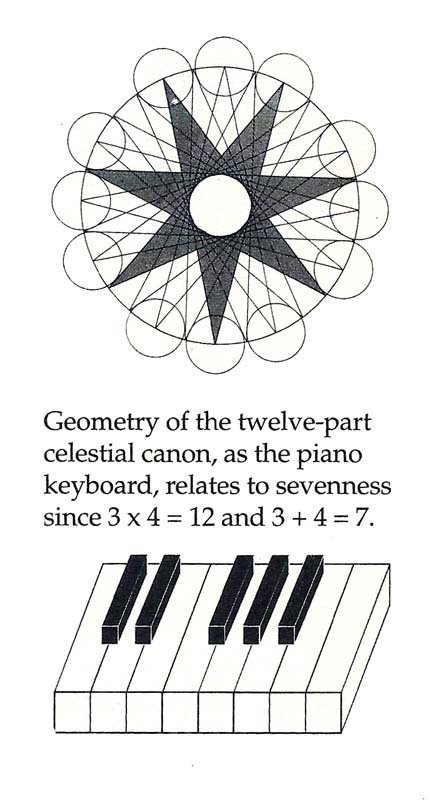Credit: Michael Schneider – A Beginner’s Guide to Constructing the Universe

The Diatonic Scale

These are the 7 notes used from time immemorial.  The 8th note is the return of the Octave.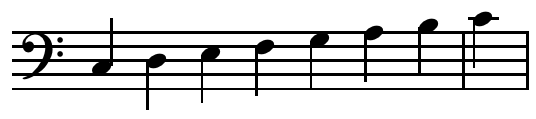In ancient times, they were traditionally arranged to play the scale downward, as if descending from heaven.

Do – Dominus –          “Lord”

Si – Sider –                 “Stars”

La – Lactea –              “Milk”

So – Sol –                    “Sun”

Fa – Fata –                  “Fate”

Mi – Microcosmos –   “Small universe”

Re – Reginia Coeli –   “Queen of the Heavens”

Do – Dominus –          “Lord”

“The seven-note scale is meant to model the hidden side of the macro-cosmic design, the universe ruled by mathematical harmonies of music.  The scale structure implies that the universe emerges from absolute divinity, descends through a seven-stage celestial hierarchy and returns to absolute divinity.”2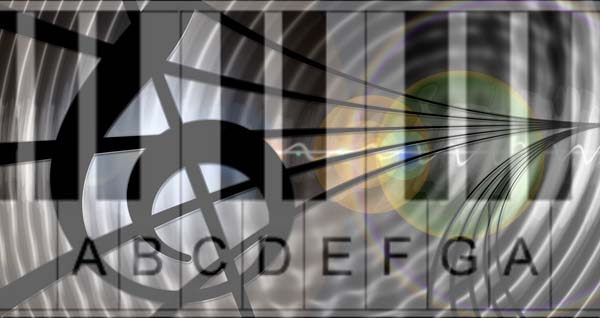As Michael Schneider writes, “Music was meant to allow the higher principles to enter our lives through our sense of hearing and our emotion.”

The Chromatic Scale

The Chromatic scale includes the 7-note diatonic scale.  It consists of 12 notes with the 13th as the return of the octave.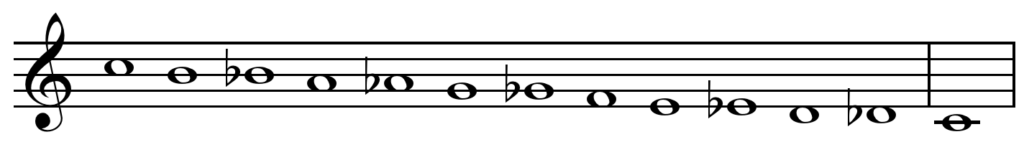It also includes 5 sharps or flats between the diatonic scale notes.

C  C#  D  D#  E  F  F#  G  G#  A  A#  B

The Pentatonic Scale

The Pentatonic scale is commonly used in Chinese, Polynesian and Scottish music.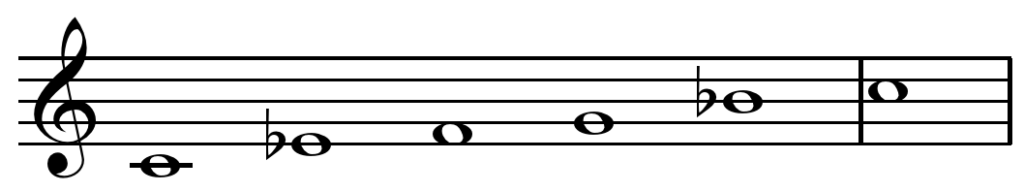It uses 5 of the 12 notes and produces the feeling of yearning.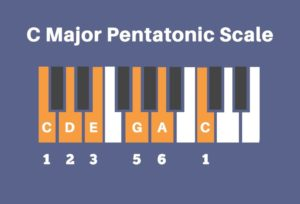Sound, Light & Color

“Light, color and sound share the same wave patterns.  What is more, they share the same vibration rates, as J. Dauven proved in 1970.  Diagram A is a composite of both vibrations; sound frequencies (the number of vibrations per second) are shown with a dash-dotted line, and color frequencies are shown with a solid line.  The closeness of the two lines indicates that the experience of harmonious rhythms is shared by the eye and the ear, even though one registers it as color, the other as sound.”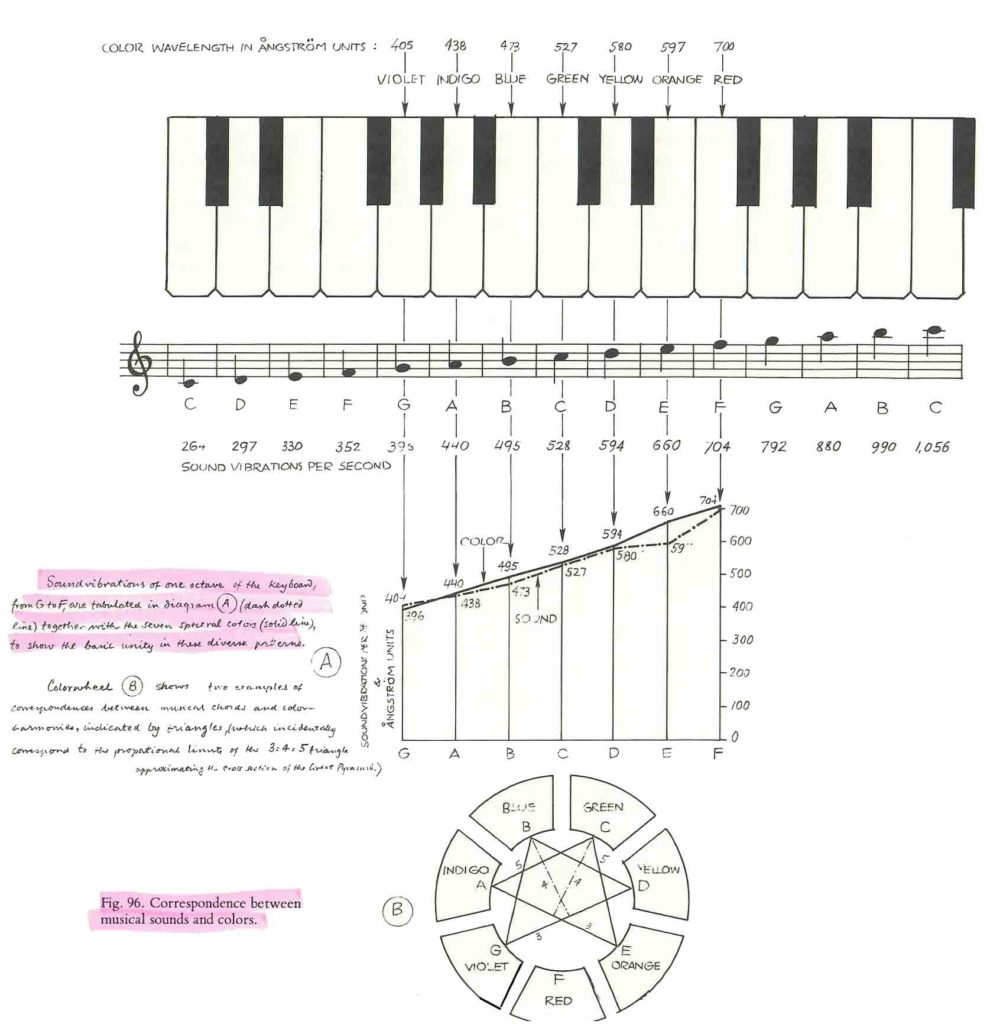Correspondence between musical sounds and colors.  Sound vibrations of one octave of the keyboard from G to F are tabulated in diagrams A together with the seven spectral colors to show the basic unity in these diverse patterns.  Credit: Gyorgy Doczi – The Power of Limits, 1981

Intervals

An interval is the distance between 2 notes in a musical scale.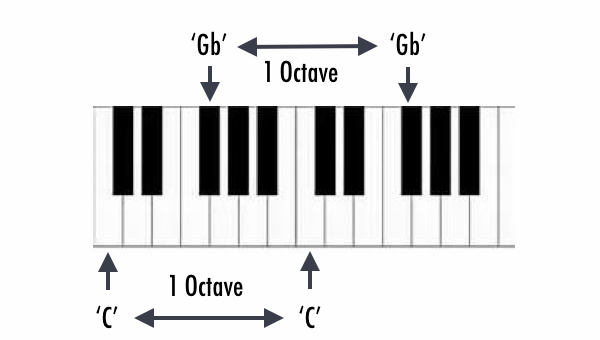For example: between A220 and A440 = 1 octave, or

between C and C# = 1 half-step (distance between 2 adjacent keys on a piano or 2 adjacent frets on a guitar).

Modern western music is based on dividing the octave into 12 half-step intervals.

To review the last article, “The 1:1 proportion, which is identity, is called unison.  The 1:2 proportion is called the octave.  The pleasant sound of the 2:3 proportion was called diapente (penta, “five”) today called the fifth, reaching through five intervals.  The consonance of the 3:4 proportion was called diatessaron (tessares, “four”) or the fourth.”

The Octave

A string length exactly half of the original will result in a tone exactly twice that of the original.

This is the concept of halving = doubling we return to again and again in Cosmic Core.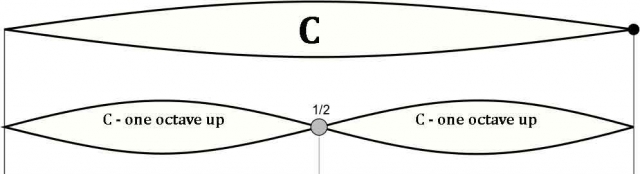½ has created its mirror opposite: 2/1.  Halving = Doubling.

It is a tangible experience of the simultaneity of opposites.

It is an auditory perception of a simultaneous interwovenness of interior with exterior.

It will create the same note, but one octave up.

“An octave sound has the strange characteristic that it is of the same quality as the fundamental tone, so much so that it seems to blend into it, yet it is definitely higher in pitch.”3

The octave creates the tone of concord.

It is the foundation upon which to build the musical scale.

Robert Lawlor tells us, “For Pythagorean thinking, the sounding of the octave (an octave is for example two successive ‘do’s’ on a musical scale) was the most significant moment of all contemplation.  It represented the beginning and goal of creation…  It is the same note, yet it is different; it is the completion of a cycle, a spiral from seed to new seed.  This timeless, instantaneous recognition (more accurate than any visual recognition) is universal among humans.”4

He goes on to say, “The experience of hearing the octave contains the mystery of a simultaneous sameness and difference.  This quality of perceiving both sameness and difference is part of the poise of mind that sacred geometry means to cultivate: one which is precisely discerning yet harmoniously integrating.”5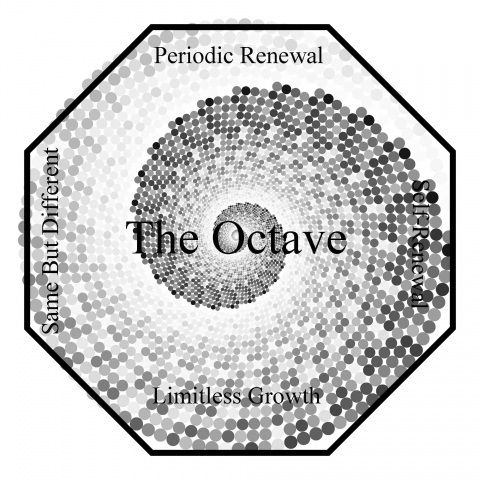Octave Formula

f = 2n * f0

f0 – fundamental frequency

f – same note in higher or lower octave

n – an integer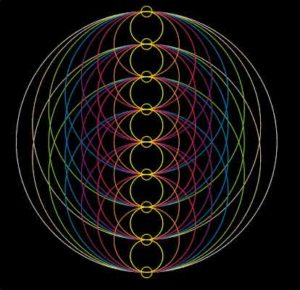Geometry of the Octave

Daipason, the octave, has the 1:2 = 0.5 proportion of a rectangle composed of two equal squares, having a diagonal of 5 length, which is the joint length of two reciprocal golden rectangles.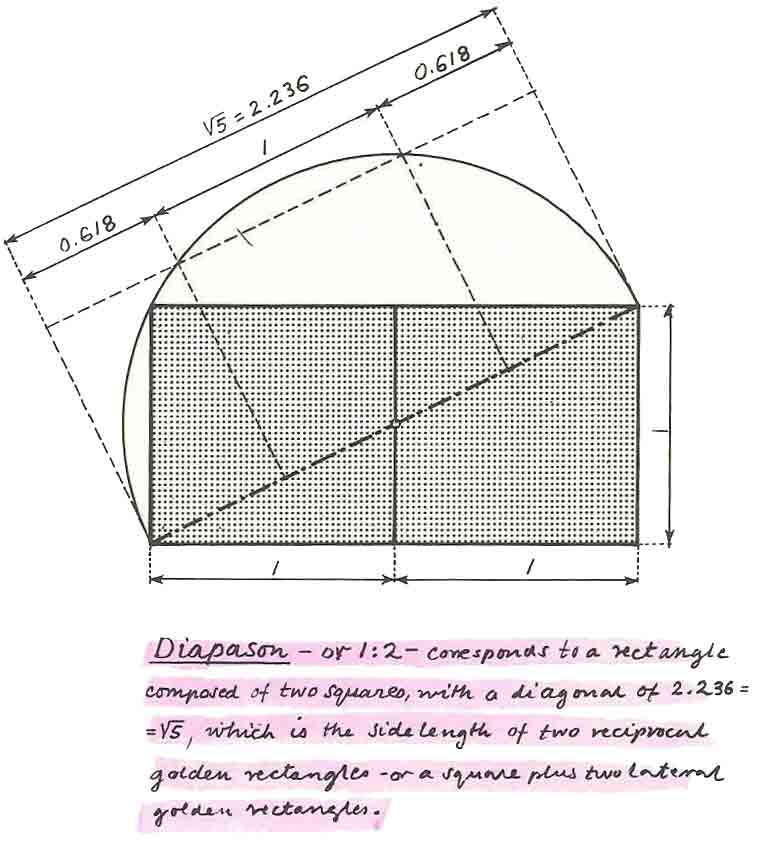Credit: Gyorgy Doczi – The Power of Limits, 1981

Geometry of One Octave (Halves)

The octave can be expressed geometrically in various ways.

An equilateral triangle in a circle defines the octave as its circumcircle is twice the diameter of its incircle.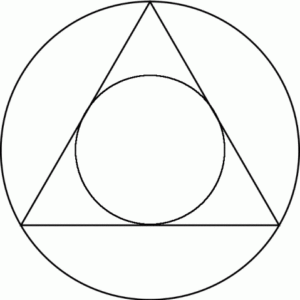The circumcircle (outer circle) is twice the diameter of the incircle (inner circle).  This creates the ratio 2:1 – the octave.

The yin yang Taoist symbol expresses the octave in a profound way.  There are two equal circles within the larger circle.  The diameter of each smaller circle is exactly ½ that of the larger circle.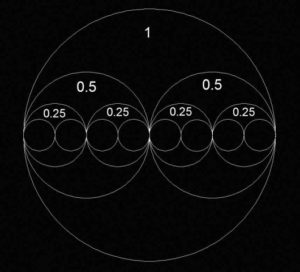Geometry of Two Octaves (Quarters)

The geometry of two octaves can also be expressed geometrically.

This is seen in:

• A circle within a triangle within a larger triangle – two triangles
• Decomposed squares – Four squares
• A pentagon in a pentagram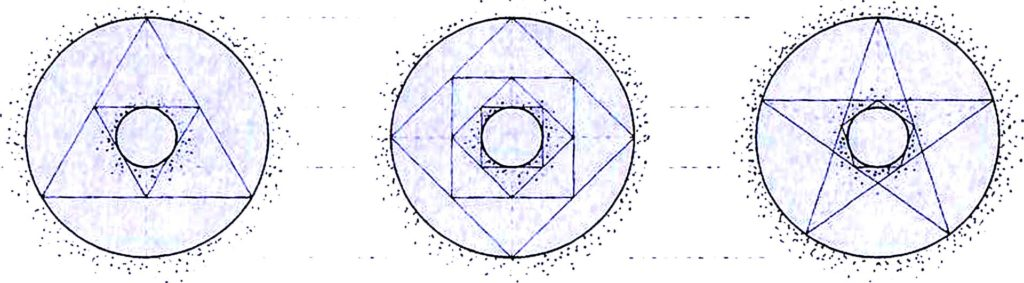Credit: page 84 – Quadrivium

Octave-shifting

Cosmic frequencies, like very low frequencies of planetary motions can be octave-shifted, or multiplied by 2 many times over to get the same note in the audible range of frequencies.

For example:

Earth frequency = 1 cycle per year

1 year = 31,556,926 seconds

Earth Frequency = (1/31,556,926) Hz = .000000031556926 Hz

Multiply by 2, 36 times and get 136.1 Hz

136.1 Hz is C#, the note of the Earth year, 36 octaves higher.

The Fifth

Holding a string at its 2/3 length creates the fifth – that is the fifth note ahead in the musical sequence.  This is seen as the red dot below.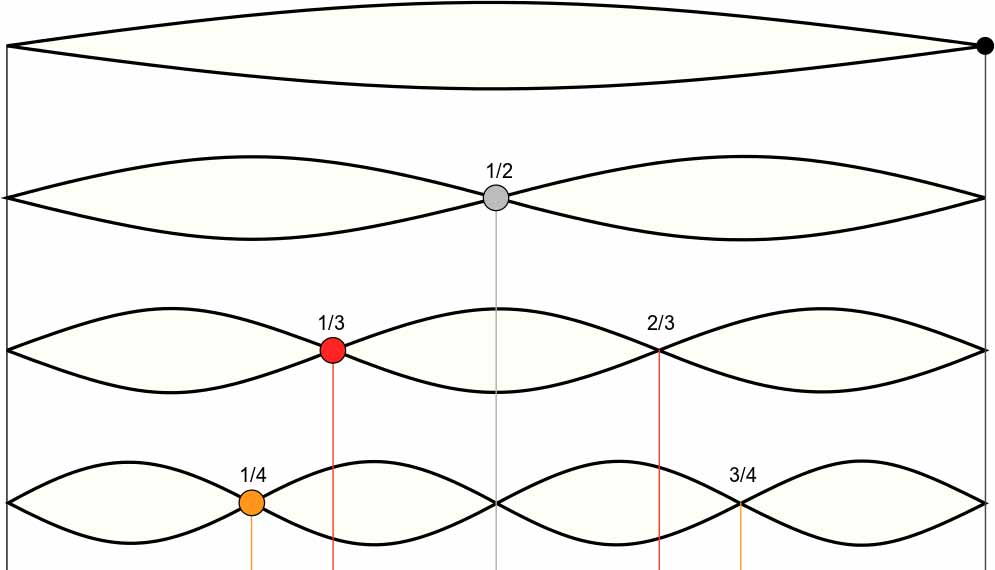The fifth is the “chord of triumph” – a beautifully consonant sound.

One part is twice as long as the other.  This produces another octave.

The Geometry of the Fifth

The 2:3 = 0.666 proportion of diapente is a close approximation of the golden section’s 0.618 ratio.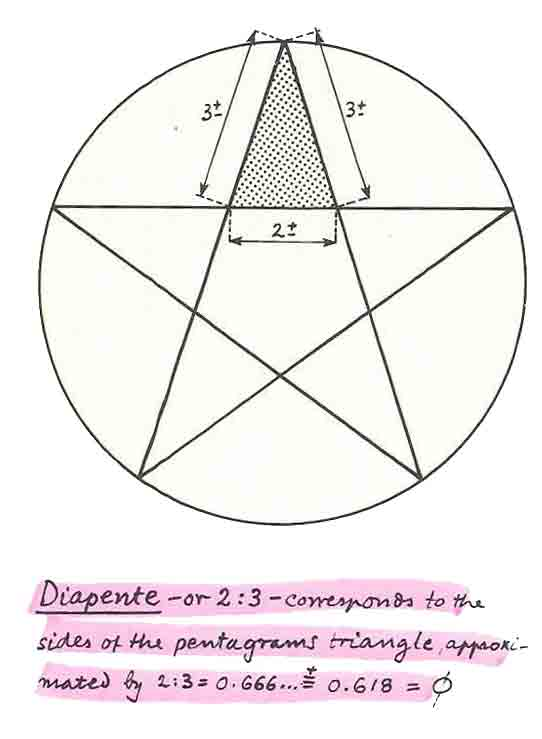Credit: Gyorgy Doczi – The Power of Limits, 1981

The “Circle of Fifths”

The circle of fifths is more appropriately called the “Spiral of Fifths”.

It spirals clockwise continually dividing each 2/3 section by another 2/3.

This creates a sequence of shorter strings and higher tones.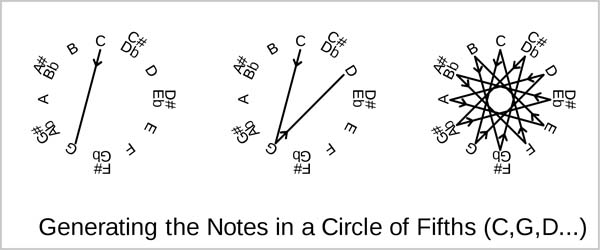This builds upon and maintains the same harmonious relationship with the notes before and after.

After the 7th division the notes repeat the earlier cycle, but slightly sharp.

After the 12th division the original note is virtually sounded again, but slightly flat.

12 tones (chromatic scale) then spread across 7 octaves.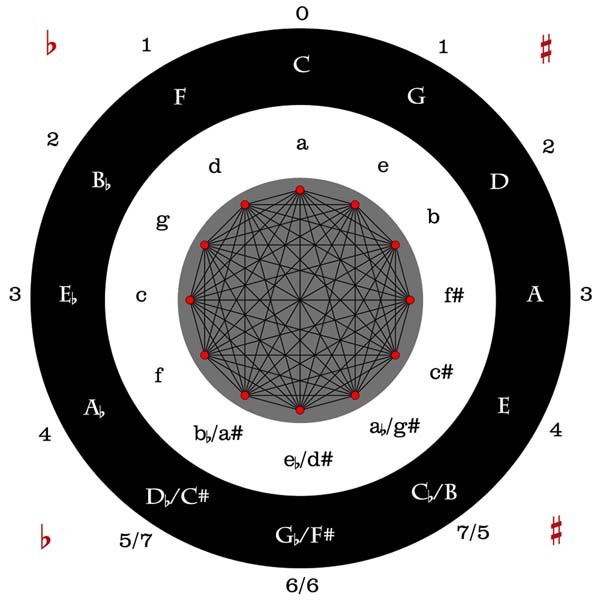The Circle of Fifths

Each subsequent division produces the fifth note ahead in the musical sequence A-B-C-D-E-F-G.

Whole note A at 2/3 produces E.

2/3 of E produces B.

2/3 of B produces F…etc.

“The spiral of fifths represents the zodiacal year in twelve phases, twelve processes of life, twelve spiritual attributes.”6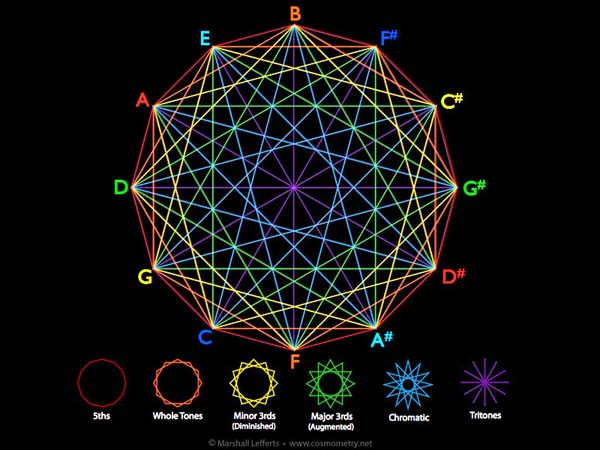Credit: Marshall Lefferts – www.cosmometry.net

The Fourth

The fourth occurs when you divide a string at ¾ its total length.  This is seen with the orange dot below.

This produces the 4th note ahead in the scale.3/4 of A produces D.

3/4 of E produces A.

3/4 of B produces E…etc.

Geometry of the Fourth

Diatessaron is identical with the 3:4 proportion of the Pythagorean triangle.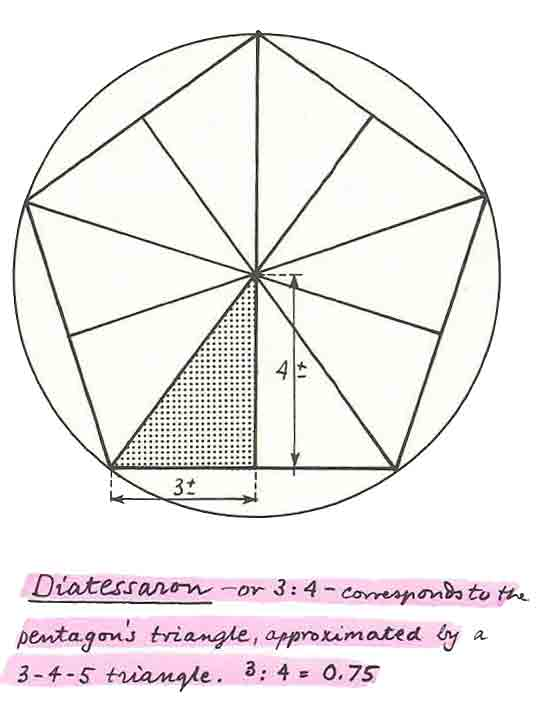Credit: Gyorgy Doczi – The Power of Limits, 1981

Spiral of Fourths

The spiral of fourths is similar to the spiral of fifths, but moves in the opposite direction.

Continually subdividing into ¾ produces the same 12 tone scale as the spiral of fifths.

The difference is that it happens less rapidly and moves in the opposite direction – counter-clockwise.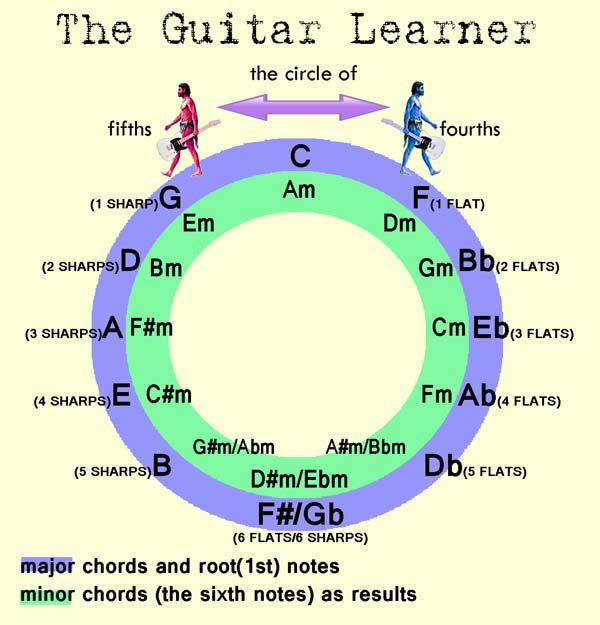A tone’s ‘fourth’ is the inverse of its ‘fifth’.

“On the spiral, the fifth note ahead is the same, but an octave different, of the fourth note behind.

A string’s fourth and its fifth added together comprise an octave, a whole comprehending both harmonies.  The tone, octave, fourth, and fifth hold the mathematics that builds the harmonious musical scale.”7

Pythagoras & Music

Pythagoras developed and taught a musical scale before 500 BC.

He supposedly passed a blacksmith shop and heard familiar harmonies in the ringing tones of the hammers on the anvil.

He then found the weights were responsible for the musical notes.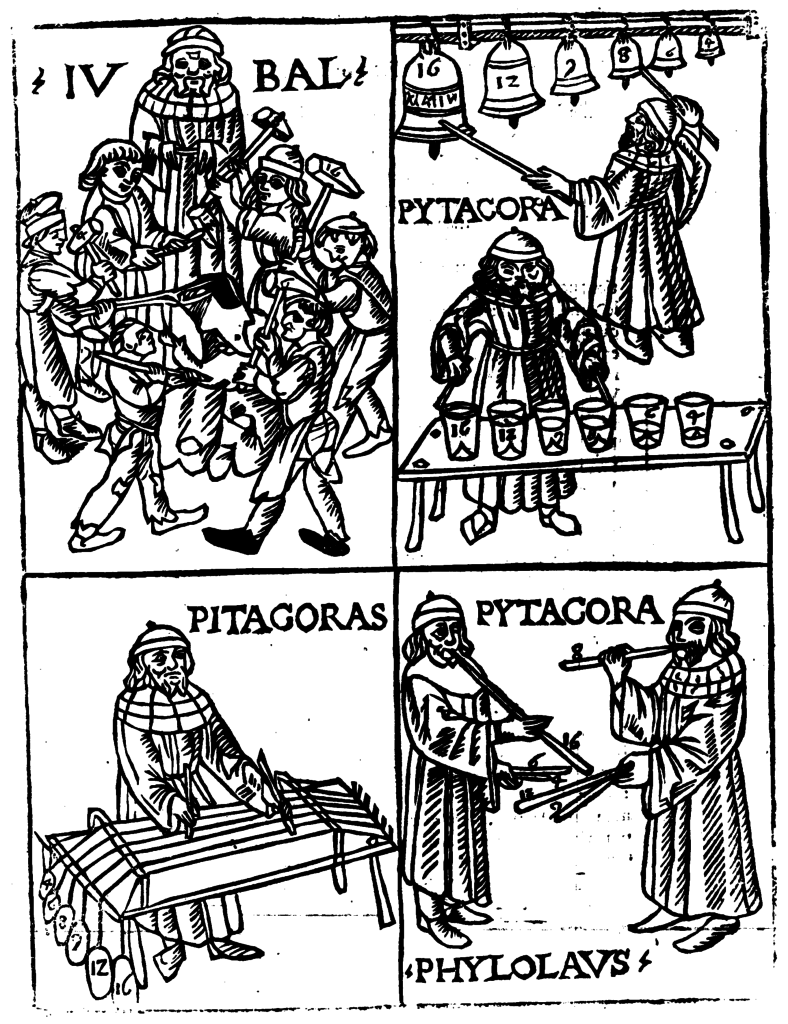A hammer that weighed ½ of another produced a sound twice as high (the octave 2:1).

A pair weighing 3:2 sounded beautiful (the fifth).

He concluded that all nature consists of harmony arising from number.  This was a precursor to the modern physicist’s assumption that nature conforms to laws expressed in mathematical form.

He was the first to teach musical principles outside of temples.

He did not invent advanced geometry, but used it in new ways, especially in regards to music.

He learned from Egypt, Chaldea & India and is said to have calmed a raging bear and soothed a drunken man by playing certain tunes on the lyre.

“Music of the Spheres”

The “music of the spheres” relates to the harmonious cosmic relationships found throughout reality on all scales and can be exemplified by the harmonic proportions among the celestial bodies such as the Sun, Moon, Earth and other planets.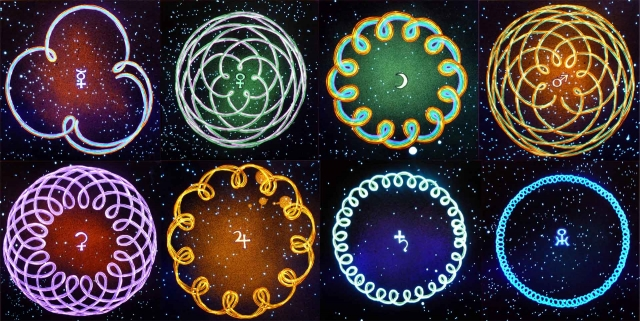Planetary orbits of Earth & Mercury, Venus, the Moon, Mars, Ceres, Jupiter, Saturn, Uranus

The point is that music is based on the mathematical relationships found in nature.

These can be expressed by ratios of simple whole numbers.  For instance:

Earth & Venus = 8:13

Neptune & Pluto = 3:2 (Perfect Fifth)

Jupiter’s Moon Europa to its Moon Ganymede = 2:1 (Perfect Octave)

Saturn’s Moon Enceladus and its Moon Dione = 2:1 (Perfect Octave)

Saturn’s Moon Titan and its Moon Hyperion = 4:3 (Perfect Fourth)

Saturn’s Moon Mimas and its Moon Tethys = 2:1 (Perfect Octave)

Earth & Moon = 1:1 (Perfect Unison)

Visit here for visuals.

Pythagorean Tuning – 432 Hertz

432 Hz sounds brighter, very powerful, enchanting and therapeutic.

This tuning led to the modern day musical scale.

Within this tuning all musical notes are found by using mathematics.

It is based on fifths.

Pythagoras gave number values according to their placement on a master grid.

He started at note 1 and used fifths.

Through mathematics he was guided to note 27 (hertz).

He kept doubling to produce 54, 108, 216, 432…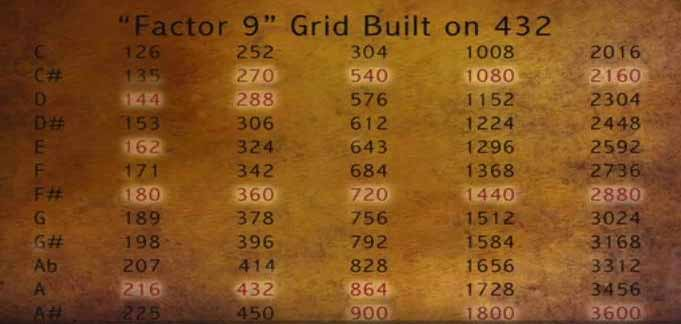The 13th step should bring you back to the starting note seven octaves later.

It is actually flat.

It is 98.65603…% of the ideal.

This difference is called the “Comma of Pythagoras”.

Equal-Tempered Scale – 440 Hertz

The equal-tempered scale was developed in the 17th century so orchestras could tune their instruments precisely with each other.

The comma of Pythagoras was distributed evenly between the 12 notes.

Each note was thus flattened by 1/48.

The 13th tone will now match the 1st tone, but an octave higher.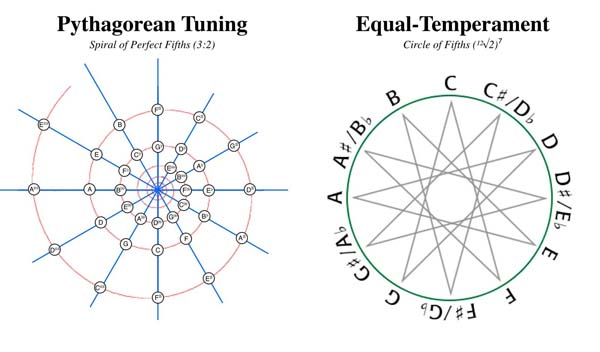According to many, this saps the power and therapeutic value in the tiny differences because the harmonies do not align with the natural harmonies of reality.

There exists an interesting debate in the music community regarding this.  One must come to their own conclusions.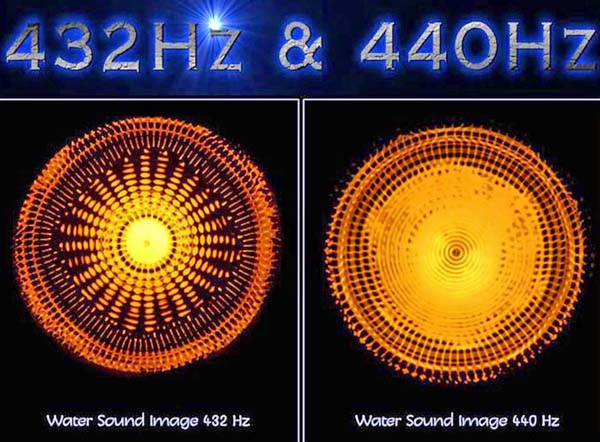432

432 is very important in Pythagorean tuning.

The numerical value of a frequency is dependent on the length of one second in time, which, it is argued, is arbitrary.  However, it is not arbitrary if you base it on astronomical observations and cosmological cycles as the ancients did.  One second was an exact division of the amount of time it took the Earth to make one complete revolution around the Sun.

Interestingly, in 1967 the second began to be defined in terms of the natural periodicity of the radiation of a cesium-133 atom.  The International Committee of Weights and Measures announced in 1967, “The second is the duration of 9,192,631,770 periods of the radiation corresponding to the transition between the two hyperfine levels of the ground state of the caesium-133 atom.”

They changed the definition at this time “to improve the stability of timekeeping in a manner that would be invisible to the general population.”8

Note that the second is not a ‘length of time’ per se.  It is a ‘specific division of a larger, precise cycle’.

Furthermore, if you tune a musical instrument to A = 432 Hertz (Hz), all the other notes will be integer (whole) numbers.  If you tune to another frequency, such as A = 440 Hz, every other note is a fractional number.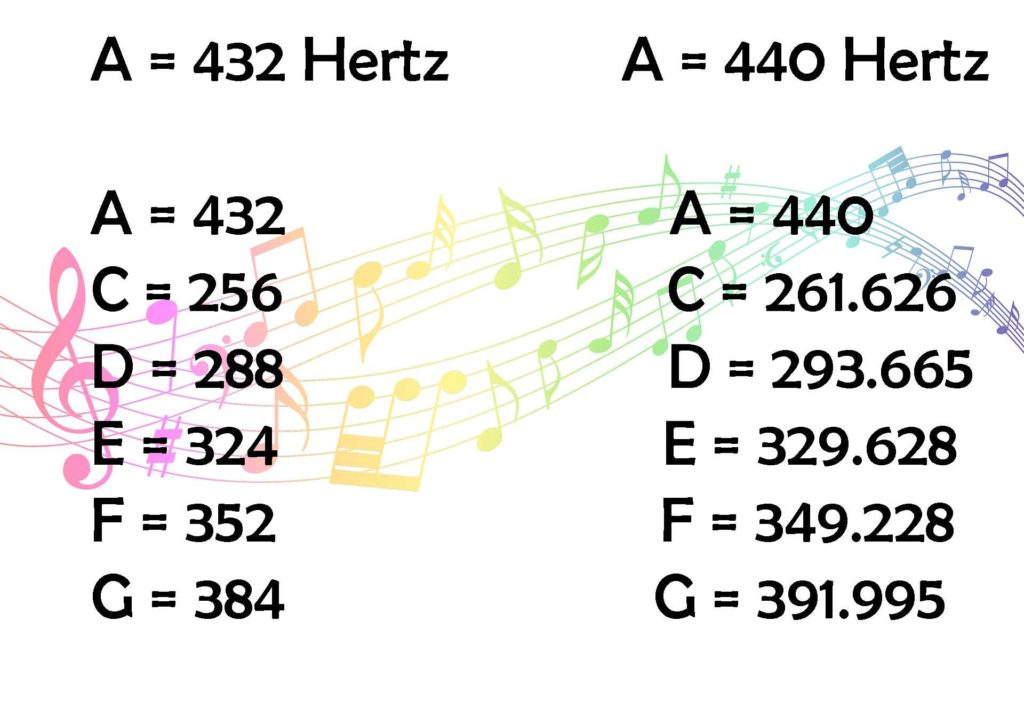Notice how one system inherently contains more harmony than the other.

This does not mean one is “better” than the other per se.  It means one is more harmonious and synched up to greater universal cycles (the year & Great Year).  It is amusing that this is such a contentious subject for many.  Take from it what you will.

Pythagoras was not calculating cycles to find 432, he just happened to find it by geometry.

Many ancient musical instruments (singing bowls…etc.) happen to produce the same tone – 432 cycles per second.

Geometry of Sound

We will take a look at the ‘sound’ of shapes.  Each shape has a number according the sum of their angles.  When these numbers are expressed in Hertz, very interesting things occur.

View Sonic Geometry to better understand these concepts.

Polygons:

Circle = 360° (sum of angles = hertz)

Square = 360°

Triangle = 180°

Pentagon = 540°

All these add up to 9 just like 432.

180 hertz = sound of a triangle = F#

360 hertz = sound of square or circle = higher F# (perfect octave up from triangle)

540 hertz = harmonic fifth of the other two = C#

720 hertz = hexagon = F#

900 hertz = heptagon = A# the note required to complete a F# major chord in perfect harmony

1080 hertz = octagon = C#

Geometry is expressed by tones.

The above frequencies produce a perfect 3 part major chord in the key of F#.

Platonic Solids: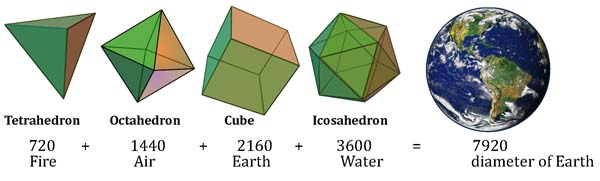Tetrahedron = 720 Hz = F#

Cube = 2160 Hz = high C#

Octahedron = 1440 Hz = higher F#

Icosahedron = 3600 Hz = A#

The A# of the icosahedron completes another F# major chord.

Seed of Life: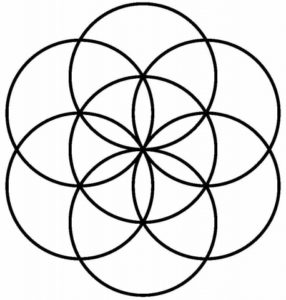1st circle = 360 degrees = F#

2nd circle = 720 = F#

3rd circle = 1080 = harmonic 5th of C#

4th circle = 1440 = F#

5th circle = 1800 = A# provides the harmonic 3rd tone of F# major chord

6th  circle = 2160 = another C#

432 vs. 440

432 is based on a “Factor-9” Grid.

9 is the sum of every note on the grid.

9 is the number required to move up or down on the grid.

We find all the geometric numbers on the grid.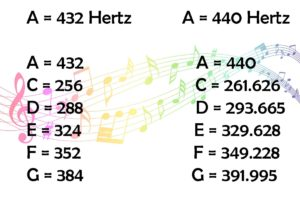440 does not have any geometric numbers.

It was adopted in 1953.  Again, draw your own conclusions about the use of 440 Hz.

2160 & 432

The cube = 2160º.

As we know 2160 = 1 Great Month (1 Zodiacal Age) in the 25,920 Great Year (Precession of the Equinoxes.

2160 is also the diameter of the Moon in miles.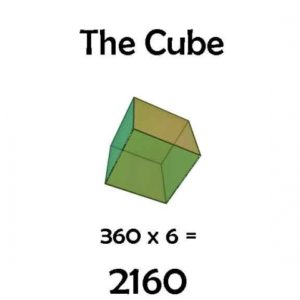432/2 = 216

2160/2 = 1080 = octagon

2160/3 = 720 = hexagon

2160/4 = 540 = pentagon

2160/5 = 432

2160/6 = 360 = square and circle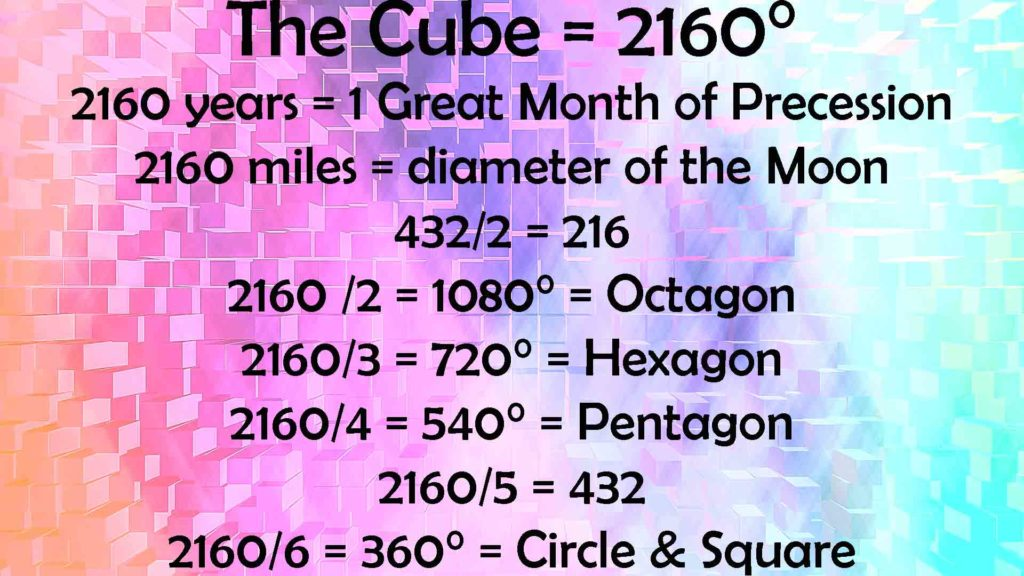The diameter of the Sun = 864,000 (432000 x 2)

There are 864,000 seconds in a day or

432,000 seconds of light (day)

432,000 seconds of darkness (night)

4322 = 186,624 miles/second (speed of light)

The accepted value for the speed of light is 186, 282 miles/second.  432 the only whole number when squared comes into .01% accuracy measuring the speed of light.George van Tassel (1910-1978) Formula

George van Tassel was an aircraft mechanic and flight inspector who worked for Douglas Aircraft, Hughes Aircraft and Lockheed.Later he claimed to have been contacted by extra-terrestrials who shared various information with him in regards to healing, health, science and spirituality.

In one contact he was given the following formula:

F = 1/t

The frequency f is the number of cycles per second.  The period t is the number of seconds by cycle.

There is a hidden importance to this equation.

Frequency = 1/time

1 = 1 Great Year          (25,920 years)

Time = 60                    60 is used because it is the ‘measure’ of time.

F = 25920/60 = 432

This reveals the synchronistic nature of all life on the planet.

13, 33 & 432

144000/432 = 333.33333…

There are 13 steps of the pyramid on the dollar bill.

13 x 33.3 = 432.9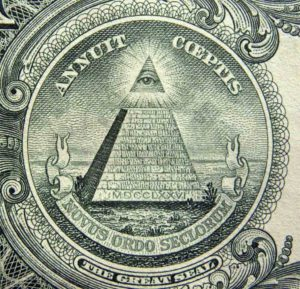The star on the dollar bill is composed of 13 smaller stars: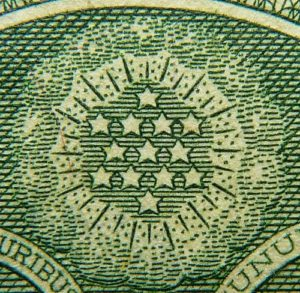This is the representation of 2 interlocking Pythagorean tetraktys: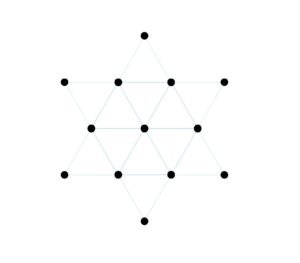Each tetraktys has four levels: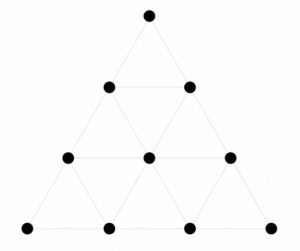4 dots at the bottom

3 dots on the second level

2 dots of the third level

The top level has a single dot representing the all-embracing unity of creation.

The flat-topped pyramid seen above on the dollar bill represents the tetraktys without its top point.  Thus is symbolizes 432.

Constructing the Ancient Scale

The ancient Pythagorean scale is found through geometry alone.

There is no need for numbers, ratios, fractions, decimals, or arithmetic.

You can construct an instrument by wrapping a guitar string or piano wire around properly placed nails in wood or a sounding box; or by hole placements for a woodwind instrument.

Reference Construction Lesson #73: Constructing the Ancient Scale.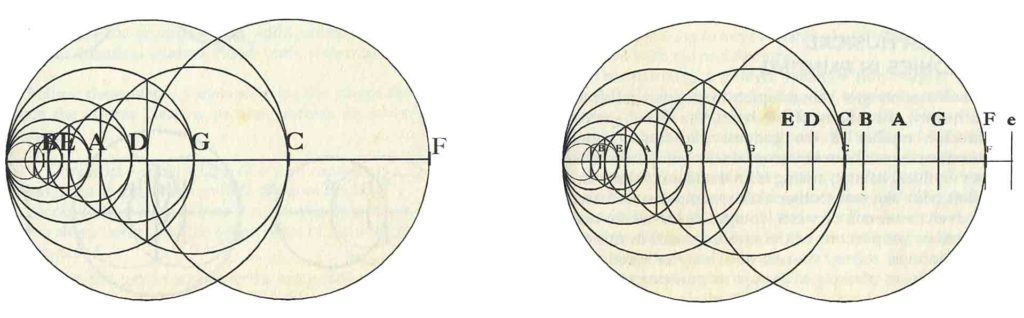Credit: Michael Schneider – A Beginner’s Guide to Constructing the Universe

“Gaze at the completed geometry until it seems to become a tunnel of expanding vesica pisces.”10

The 12 notes span 7 octaves.

To lower notes we double the short lengths.

“Every doubling of a string’s length lowers the octave but maintains the note.”11

We must double some twice and some three times.

This produces the ancient sequence of string lengths for the Pythagorean scale.1. Schneider, Michael, A Beginner’s Guide to Constructing the Universe, Harper Perennial, 1994
2. ibid.
3. Lawlor, Robert, Sacred Geometry: Philosophy & Practice, Thames & Hudson, 1982
4. ibid.
5. ibid.
6. Schneider, Michael, A Beginner’s Guide to Constructing the Universe, Harper Perennial, 1994
7. ibid.
8. Sullivan, Donald B, How does one arrive at the exact number of cycles of radiation a cesium-133 atom makes in order to define a second? Scientific American, https://www.scientificamerican.com/article/how-does-one-arrive-at-th/
9. Schneider, Michael, A Beginner’s Guide to Constructing the Universe, Harper Perennial, 1994
10. ibid.
11. ibid.